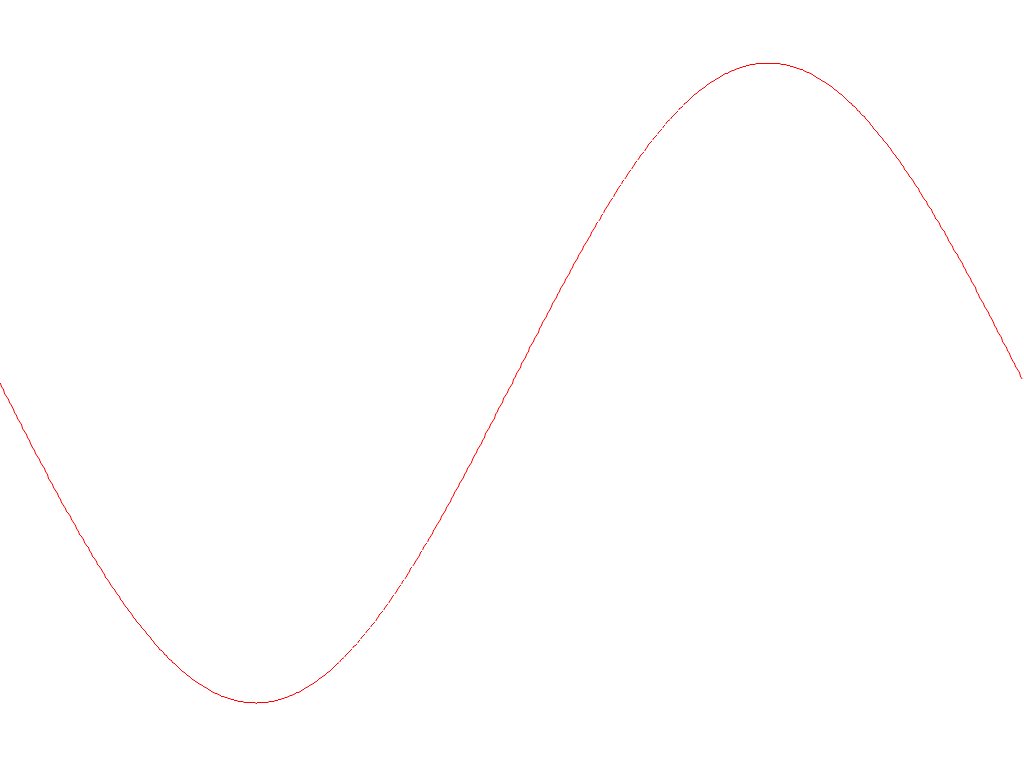# Getting Started

Let's have a quick walk through on how to produce a data plot with Plotters. This is an example that plotting the graph of a simple equation `y = sin(x)` to a PNG file.

## Step 0 - Install prerequisite libraries to system

Plotters may use some library installed on your system, depends on what operating system you are using.

• For Linux user: please make sure `libfontconfig` package is installed. For Ubuntu/Debian user, use the following command to install them.

``````sudo apt-get install libfontconfig libfontconfig1-dev
``````
• For Windows and OSX user: No prerequisite library is required.

## Step 1 - Add dependency to `cargo.toml`

In order to use Plotters, add the following line to your `cargo.tmol`

``````[dependencies]
plotters = "0.3"
``````

Alternatively if you have cargo-edit, use the following command to add the dependency

``````cargo add plotters
``````

### Step 2 - Add plotting code

As an example, we provide a minimal code that draws the function graph. We can just simply put the following code to the `src/main.rs`

``````use plotters::prelude::*;

fn main() {
let root_drawing_area = BitMapBackend::new("images/0.1.png", (1024, 768))
.into_drawing_area();

root_drawing_area.fill(&WHITE).unwrap();

let mut chart = ChartBuilder::on(&root_drawing_area)
.build_cartesian_2d(-3.14..3.14, -1.2..1.2)
.unwrap();

chart.draw_series(LineSeries::new(
(-314..314).map(|x| x as f64 / 100.0).map(|x| (x, x.sin())),
&RED
)).unwrap();
}
``````

## Step 3 - Build and run

Use the following command to build and run the example

``````cargo run
``````

And the output will be saved under the current working directory with the file name `/images/0.1.png`.